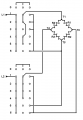# Calculating the value of in-circuit resistors

#### GS3

Joined Sep 21, 2007
408
This is inspired by another, similar ongoing thread. You do not need to read and understand the entire premise and explanation, you can go to the end and read the question which is purely mathematical.

Some time ago I had to replace a hot plate of an electric cooking stove. The plate is just a round, enclosed part with four electric terminals. After doing some tests I suspect internally it has four resistors connected as a square (or diamond if you prefer) and the four terminals are the four corners. By connecting the full voltage of the mains in different ways to different corners the heating power can be regulated.

When I installed it I was curious about determining the values of the four individual elements but I soon gave up. Note that I do not have access to the elements individually but that they are always connected inside the hot plate.Draw a square and the four sides are the resistors, R1 at the top, R2 right, R3 bottom, R4 left. The four terminals are T1 at top left, T2 top right, T3 bottom left, T4 bottom right.

The power switch has six active positions plus a seventh OFF position. Let us call the two power cables L1 and L2.

Using | to mean parallel:

In position P1:
- L1 is applied to T1 and T3 and
- L2 is applied to T2 and T4.
This puts all four elements in parallel and applies the full voltage to them.
RP1 = R1 | R2 | R3 | R4

In position P2:
- L1 to T1 and T3
- L2 to T4
RP2 = R3 | R4

In position P3:
-L1 to T3
-L2 to T4
RP3 = R3 | (R1 + R2 + R4)

In position P4:
-L1 to T1
-L2 to T4
RP4 = R4 | (R1 + R2 + R3)

In position P5:
-L1 to T1
-L2 to T3 and T4
RP5 = R4 | (R1 + R2)

In position P6:
-L1 to T3
-L2 to T4 and T2
RP6 = R3 | R2

The Question:

How can we calculate R1, R2, R3, R4 as a function of RP1 to RP6?

We have six equations. Four should be enough and the other two should be in agreement and serve to confirm the results.

RP1 = R1 | R2 | R3 | R4
RP2 = R3 | R4
RP3 = R3 | (R1 + R2 + R4)
RP4 = R4 | (R1 + R2 + R3)
RP5 = R4 | (R1 + R2)
RP6 = R3 | R2

#### #12

Joined Nov 30, 2010
18,224
Your basic problem is that the resistance elements change resistance significantly when heated, often by as much as ten to one. Cold resistance is very different from hot resistance. You can measure the voltage and current to derive the hot resistance and ignore power factor corrections because there is no inductive or capacitive reactance.

#### GS3

Joined Sep 21, 2007
408
Your basic problem is that the resistance elements change resistance significantly when heated, often by as much as ten to one. Cold resistance is very different from hot resistance.
That is not correct. The resistance varies very little. I have measured it.

Checking the internet I see Nichrome wire, typically used in heaters, when heated from room temperature to 315 ºC increases resistance by 3.3%. I am guessing the hot plate does not reach that temperature so let's say resistance increases by 2.5%. That deviation is within the tolerance of high precision resistors. And probably any measurements I make are in that range of error anyway.

In any case, I am just trying to work out the set of equations without too much focus on the specific actual values.

#### wayneh

Joined Sep 9, 2010
17,471
This is an algebra problem and I'm sure you can do it for yourself. You just need to know how to add resistances in parallel and in series, which I suspect you already know. The rest is just brute force.

Or you could just use a spreadsheet and iterate until it converges on a solution.

#### #12

Joined Nov 30, 2010
18,224
That is not correct. The resistance varies very little. I have measured it.
If you already know about how much the resistance changes, why are you on an electronics site to do algebra?